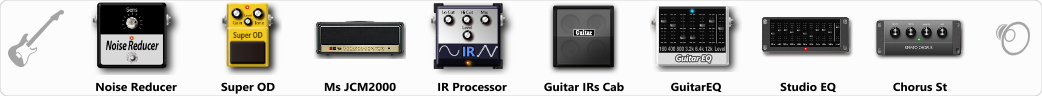# MS JCM 2000

Discussion in 'ToneLib-GFX presets' started by Shatarov Igor, Feb 4, 2020.

1. MS JCM 2000

Preset name: Mesa

Effects chain:Effect: "Noise Reducer" (Dynamics / Filter), active - "yes"
"Sens" = 72
"Mode" = Hard

Effect: "Super OD" (Overdrive / Distortion), active - "yes"
"Drive" = 62
"Tone" = 65
"Level" = 91

Effect: "Ms JCM2000" (Amp simulators), active - "yes"
"Gain" = 40
"Bass" = 93
"Middle" = 34
"Treble" = 63
"Presence" = 76
"Master" = 49
"Output" = 50
"Level (dB)" = 6

Effect: "IR Processor" (Dynamics / Filter), active - "yes"
"IR" = BBIRD 9100A 1960A P3_dc
"Low Cut (Hz)" = 151
"Hi Cut (kHz)" = 17.5
"Mix" = 100
"Level (dB)" = 0

Effect: "Guitar IRs Cab" (Cabinets), active - "yes"
"Model" = Hiwatt Fane (4x12")
"Mic Position" = Center
"Mic Distance" = Near
"Low Cut (Hz)" = 164
"Hi Cut (kHz)" = 20.0
"Mix" = 100
"Level (dB)" = 2

Effect: "GuitarEQ" (Dynamics / Filter), active - "yes"
"160 Hz" = 0
"400 Hz" = 0
"800 Hz" = -2
"1.6 kHz" = -2
"3.2 kHz" = 0
"6.4 kHz" = -1
"12 kHz" = 0
"Level (dB)" = 2

Effect: "Studio EQ" (Dynamics / Filter), active - "yes"
"31 Hz" = 0
"62 Hz" = 0
"125 Hz" = -1
"250 Hz" = -3
"500 Hz" = -5
"1 kHz" = 1
"2 kHz" = 3
"4 kHz" = 3
"8 kHz" = 2
"16 kHz" = -2
"above 16 kHz" = 0
"Level (dB)" = 0

Effect: "Chorus St" (Modulation / Sfx), active - "yes"
"Speed" = 1.9
"Depth" = 31
"Center" = 4.4
"Mix" = 64

Note: You will need to download and install the ToneLib-GFX software to use the preset.

#### Attached Files:

• ###### MS_JCM_2000.tlgfx
File size:
54.8 KB
Views:
3,198
SEMENOV, emre, agamenondsl and 4 others like this.